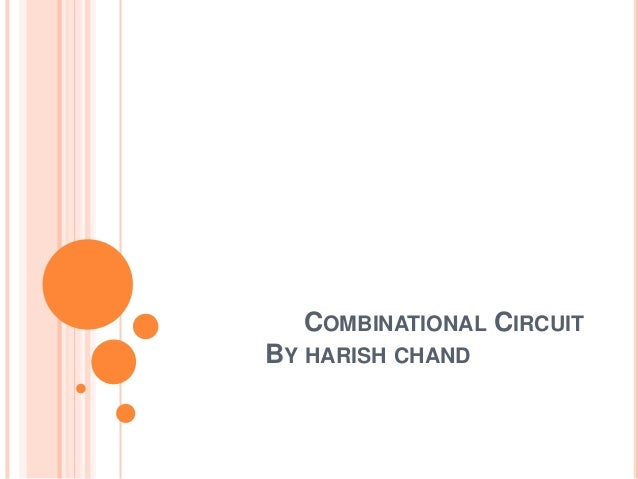Successfully reported this slideshow.
We use your LinkedIn profile and activity data to personalize ads and to show you more relevant ads. You can change your ad preferences anytime.Upcoming SlideShare
Loading in …5
×

# Combinational circuit

Combinational circuit, Half Adder,Full Adder, Half Subtractor, Full Subtractor, Multiplexers , Demultiplexer , Decoder , Encoder

• Full Name
Comment goes here.

Are you sure you want to Yes No
Your message goes here• Login to see the comments

### Combinational circuit

1. 1. COMBINATIONAL CIRCUIT BY HARISH CHAND
2. 2. OVERVIEW:  Combinational circuit is a circuit in which we combine the different gates in the circuit for example encoder, decoder, multiplexer and demultiplexer.  Combinational circuit consists of logic gates whose outputs depend on the present inputs.
3. 3.  Some of the characteristics of combinational circuits are following:  The output of combinational circuit at any instant of time, depends only on the levels present at input terminals.  The combinational circuit do not use any memory. The previous state of input does not have any effect on the present state of the circuit.  A combinational circuit can have a n number of inputs and m number of outputs.  They have no memory element.
4. 4. HALF ADDER  Half adder is a combinational logic circuit with two inputs and two outputs. The half adder circuit is designed to add two single bit binary numbers A and B. It is the basic building block for addition of two single bit numbers. This circuit has two outputs carry and sum.
5. 5. FULL ADDER  Full adder is developed to overcome the drawbacks of Half Adder circuit.  It can add two one-bit numbers A and B, and carry C.  The full adder is a three input and two output combinational circuit.
6. 6. HALF SUBTRACTOR  Half subtractor is a combination circuit with two inputs and two outputs (difference and borrow).  It produces the difference between the two binary bits at the input and also produces a output (Borrow) to indicate if a 1 has been borrowed. In the subtraction (A-B), A is called as Minuend bit and B is called as Subtrahend bit.
7. 7. FULL SUBTRACTOR  The disadvantage of a half subtractor is overcome by full subtractor.  The full subtractor is a combinational circuit with three inputs A,B,C and two output D and C'. A is the minuend, B is subtrahend, C is the borrow produced by the previous stage, D is the difference output and C' is the borrow output.
8. 8. MULTIPLEXERS  Multiplexer is a special type of combinational circuit. There are n-data inputs, one output and m select inputs with 2m = n. It is a digital circuit which selects one of the n data inputs and routes it to the output. The selection of one of the n inputs is done by the selected inputs.  Depending on the digital code applied at the selected inputs, one out of n data sources is selected and transmitted to the single output Y. E is called the strobe or enable input which is useful for the cascading. It is generally an active low terminal, that means it will perform the required operation when it is low.
9. 9. Multiplexer come in multiple variations: • 2 : 1 multiplexer • 4 : 1 multiplexer • 16 : 1 multiplexer • 32 : 1 multiplexer
10. 10. DEMULTIPLEXER  A demultiplexer performs the reverse operation of a multiplexer i.e. it receives one input and distributes it over several outputs.  It has only one input, n outputs, m select input. At a time only one output line is selected by the select lines and the input is transmitted to the selected output line.
11. 11. Demultiplexer come in multiple variations:  1 : 2 demultiplexer  1 : 4 demultiplexer  1 : 16 demultiplexer  1 : 32 demultiplexer
12. 12. DECODER  A decoder is a combinational circuit that has n input and to a maximum m = 2n outputs.  Decoder is identical to a demultiplexer without any data input.  It performs operations which are exactly opposite to those of an encoder.
13. 13. ENCODER  Encoder is a combinational circuit which is designed to perform the inverse operation of the decoder. An encoder has n number of input lines and m number of output lines.  An encoder produces an m bit binary code corresponding to the digital input number. The encoder accepts an n input digital word and converts it into an m bit another digital word.
14. 14. Thanks For Listening… 